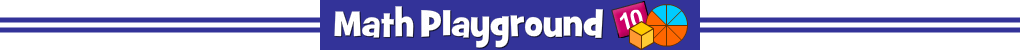AdvertisementThinking Blocks Ratios - Learning Connections
Essential Skills
Problem Solving - model and solve word problems

Common Core Connection for Grades 6 and 7
Understand the concept of ratio and describe the relationship between two quantities.
Use ratio and rate reasoning to solve real-world and mathematical problems.
Recognize and represent proportional relationships between quantities.
Use proportional relationships to solve multistep ratio and percent problems.
More Math Games to Play